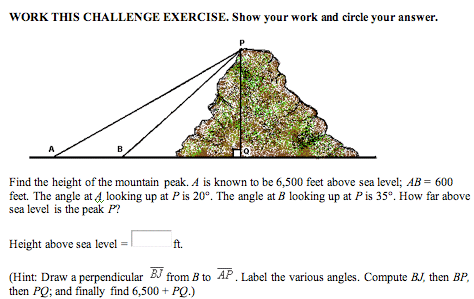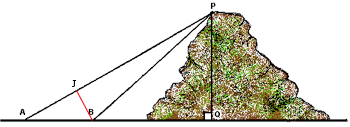SEARCH HOMEMath Central Quandaries & QueriesHello, Can you please help us (my son Jim geometry) out with this problem, we cannot seem to find the correct method of solving, not sure how to solve the scalene triangle with what is given...if that's the correct method?? Can you please explain how this is solved!! Thank you so much, Jim and son !Jim,

I followed the first hint.Since angle BJA is a right angle and the measure of angle is 20o you can use the sine function to find the length of BJ. You also know the measure of the angle ABJ and hence the measure of the angle JBP.

Can you now find the length of BP?

HarleyMath Central is supported by the University of Regina and The Pacific Institute for the Mathematical Sciences.